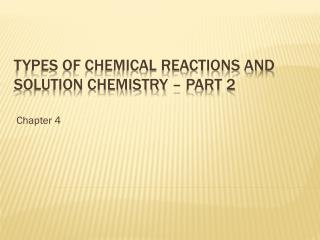DownloadDownload PresentationTypes of Chemical Reactions and solution chemistry – part 2

# Types of Chemical Reactions and solution chemistry – part 2

Download Presentation## Types of Chemical Reactions and solution chemistry – part 2

- - - - - - - - - - - - - - - - - - - - - - - - - - - E N D - - - - - - - - - - - - - - - - - - - - - - - - - - -
##### Presentation Transcript

1. Chapter 4 Types of Chemical Reactions and solution chemistry – part 2

2. Definitions • Bronsted- acids are proton donors, bases are proton acceptors • Arrhenius – acids produce H+ ions in water and bases produce OH- ions in solution • Net ionic equation for acid-base reactions H+(aq) + OH-(aq)  H2O(l) • The hydroxide ion can be assumed to completely react with even a weak acid in solution. 4.8 Acid-base reactions (neutralization reactions)

3. Stoichiometry Calculations for Acid-Base Reactions • List the species present in the combined solution before any reaction occurs. • Write the balanced net ionic equation for this reaction • Calculate the moles of reactants (may need to use volume and molarity) • Determine LR if appropriate • Calculate moles of the required species • Convert to grams or volume of solution as required in problem 4.8 Acid-base reactions (neutralization reactions)

4. Acid – Base Titrations • Vocabulary • Titrant – solution of known concentration • Analyte – solution of unknown concentration • Equivalence point – point at which the amount of titrant added to analyte results in perfect neutralization • Indicator – a substance added at the beginning of the titration that changes color at the equivalence point • Endpoint – the point at which the indicator changes color 4.8 Acid-base reactions (neutralization reactions)

5. Acid – Base Titrations • Requirements for a successful titration • The exact reaction between titrant and analyte must be known • The reaction must proceed rapidly • The equivalence point must be marked accurately (select the appropriate indicator) • The volume of titrant required to reach the equivalence point must be known accurately • For acid-base titrations, the titrant should be a strong acid or a strong base 4.8 Acid-base reactions (neutralization reactions)

6. Electron Transfer (OIL RIG) • Oxidation Is a Loss of electrons • Reduction Is a Gain of electrons • Examples of redox reactions • Photosynthesis • Combustion • Oxidation of sugars, fats and proteins for energy 4.9 Oxidation-Reduction reactions (redox)

7. Characteristics of redox reactions • The oxidized substances…….. • Lose electrons • Increase oxidation state • Is the reducing agent • The reduced substances……… • Gain electrons • Decreased oxidation state • Is the oxidizing agent 4.9 Oxidation-Reduction reactions (redox)